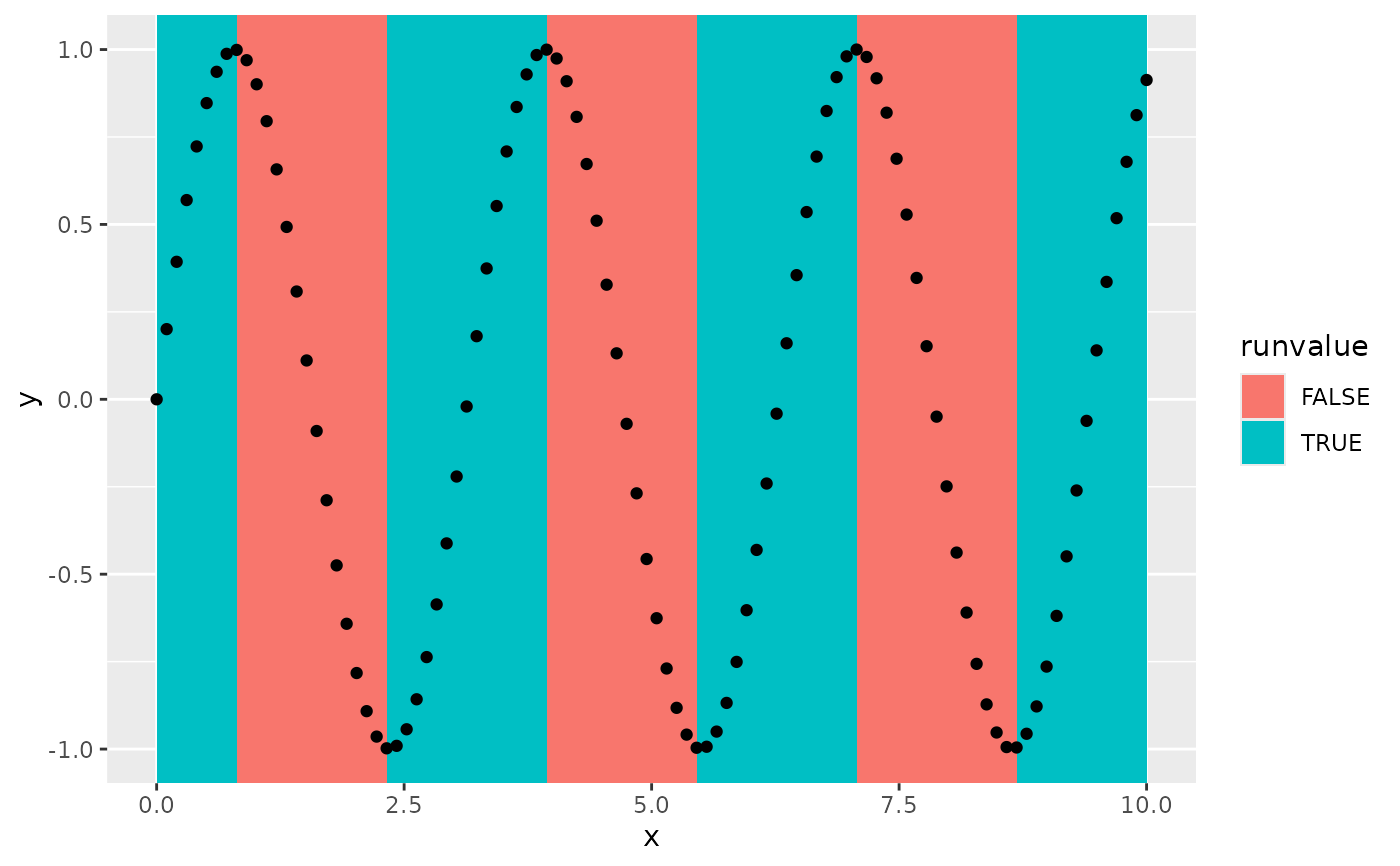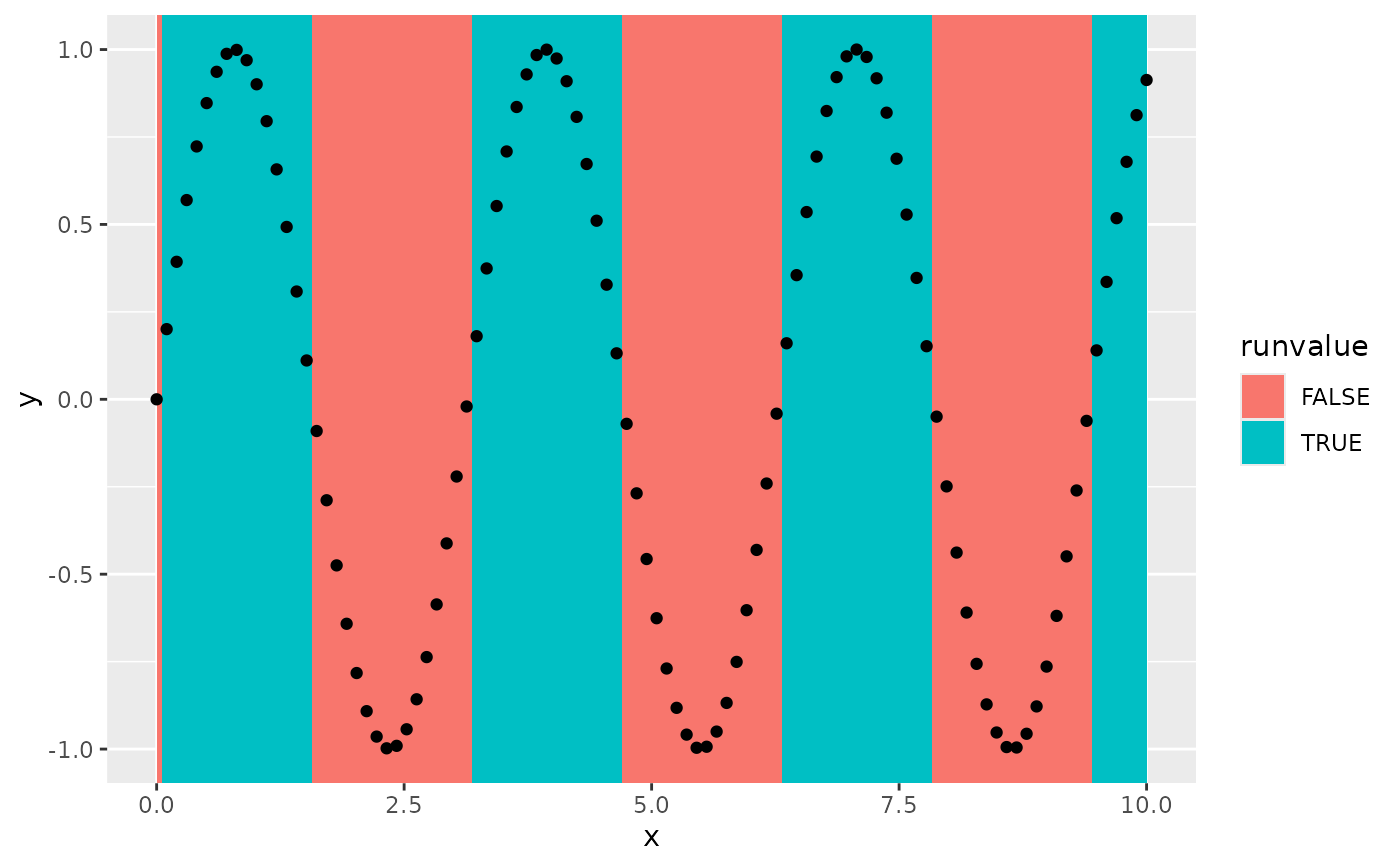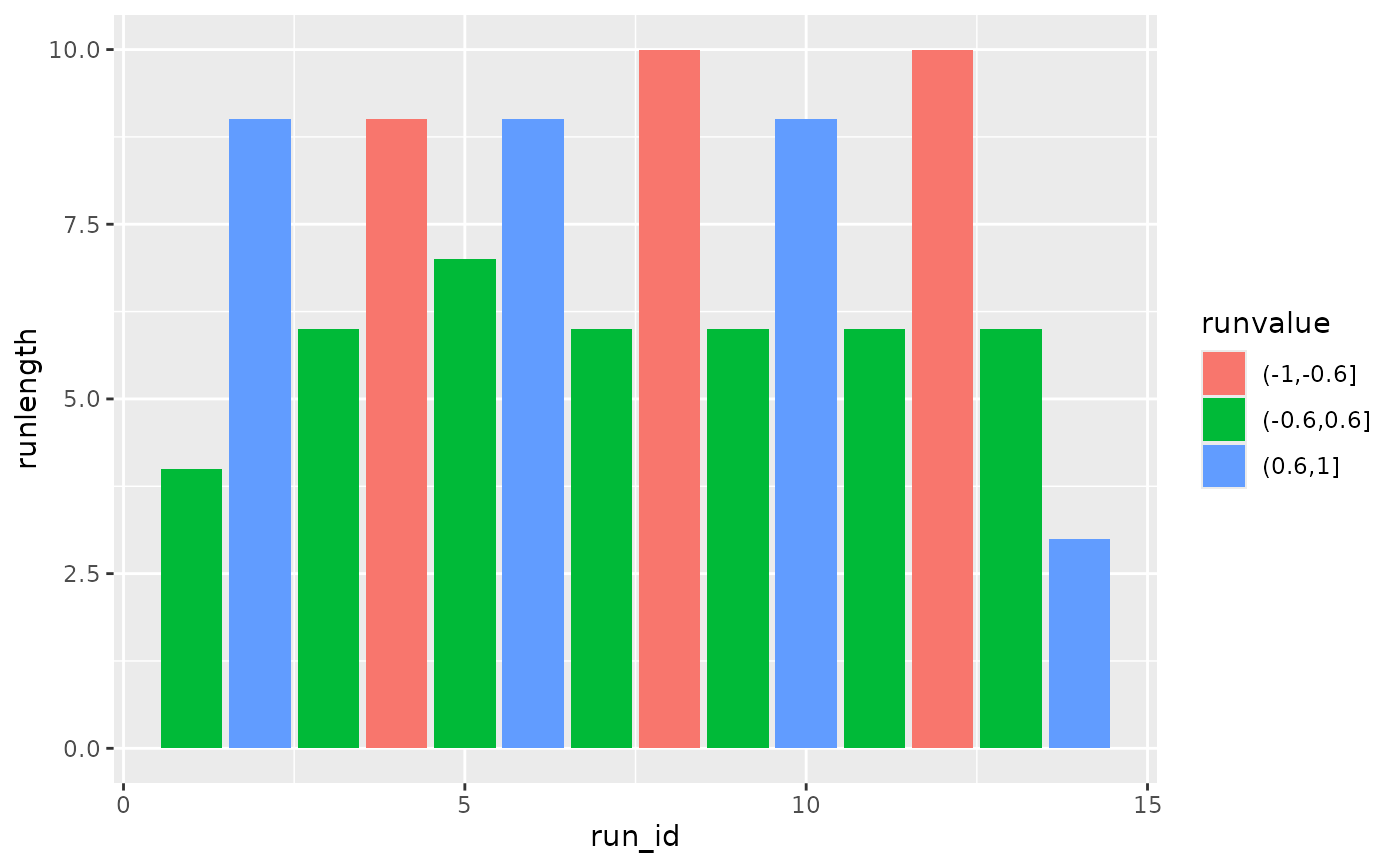Run length encoding takes a vector of values and calculates the lengths of consecutive repeated values.

## Usage

stat_rle(
mapping = NULL,
data = NULL,
geom = "rect",
position = "identity",
...,
align = "none",
na.rm = FALSE,
orientation = "x",
show.legend = NA,
inherit.aes = TRUE
)

## Arguments

mapping

Set of aesthetic mappings created by aes(). If specified and inherit.aes = TRUE (the default), it is combined with the default mapping at the top level of the plot. You must supply mapping if there is no plot mapping.

data

The data to be displayed in this layer. There are three options:

If NULL, the default, the data is inherited from the plot data as specified in the call to ggplot().

A data.frame, or other object, will override the plot data. All objects will be fortified to produce a data frame. See fortify() for which variables will be created.

A function will be called with a single argument, the plot data. The return value must be a data.frame, and will be used as the layer data. A function can be created from a formula (e.g. ~ head(.x, 10)).

geom

Use to override the default connection between geom_rect() and stat_rle().

position

Position adjustment, either as a string naming the adjustment (e.g. "jitter" to use position_jitter), or the result of a call to a position adjustment function. Use the latter if you need to change the settings of the adjustment.

...

Other arguments passed on to layer(). These are often aesthetics, used to set an aesthetic to a fixed value, like colour = "red" or size = 3. They may also be parameters to the paired geom/stat.

align

A character of length one that effect the computed start and end variables. One of the following:

"none"

Take exact start and end x values.

"center"

Return start and end x values in between an end and the subsequent start.

"start"

Align start values with previous end values.

"end"

Align end values with next start values.

na.rm

If FALSE, the default, missing values are removed with a warning. If TRUE, missing values are silently removed.

orientation

The orientation of the layer. The default (NA) automatically determines the orientation from the aesthetic mapping. In the rare event that this fails it can be given explicitly by setting orientation to either "x" or "y". See the Orientation section for more detail.

show.legend

logical. Should this layer be included in the legends? NA, the default, includes if any aesthetics are mapped. FALSE never includes, and TRUE always includes. It can also be a named logical vector to finely select the aesthetics to display.

inherit.aes

If FALSE, overrides the default aesthetics, rather than combining with them. This is most useful for helper functions that define both data and aesthetics and shouldn't inherit behaviour from the default plot specification, e.g. borders().

## Value

A ggplot2 layer

## Details

The data is first ordered on the x aesthetic before run lengths are calculated for the label aesthetic. In contrast to base::rle(), NAs are considered equivalent values, not different values.

## Aesthetics

stat_rle() understands the following aesthetics (required aesthetics are in bold)

• x

• label

• group

## Computed variables

start

The x values at the start of every run.

end

The x values at the end of every run.

start_id

The index where a run starts.

end_id

The index where a run ends.

run_id

The index of a run.

runlength

The length of a run.

runvalue

The value associated with a run.

## Examples

df <- data.frame(
x = seq(0, 10, length.out = 100),
y = sin(seq(0, 10, length.out = 100)*2)
)

# Label every run of increasing values
ggplot(df) +
stat_rle(aes(x, label = diff(c(0, y)) > 0),
align = "end") +
geom_point(aes(x, y))# Label every run above some threshold
ggplot(df) +
stat_rle(aes(x, label = y > 0),
align = "center") +
geom_point(aes(x, y))# Categorising runs, more complicated usage
ggplot(df) +
stat_rle(aes(stage(x, after_stat = run_id),
after_stat(runlength),
label = cut(y, c(-1, -0.6, 0.6, 1)),
fill = after_stat(runvalue)),
geom = "col")